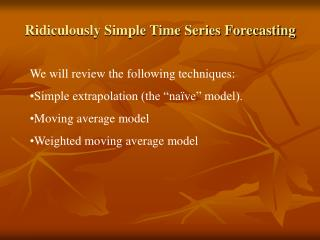DownloadDownload PresentationRidiculously Simple Time Series Forecasting

# Ridiculously Simple Time Series Forecasting

Télécharger la présentation## Ridiculously Simple Time Series Forecasting

- - - - - - - - - - - - - - - - - - - - - - - - - - - E N D - - - - - - - - - - - - - - - - - - - - - - - - - - -
##### Presentation Transcript

1. Ridiculously Simple Time Series Forecasting • We will review the following techniques: • Simple extrapolation (the “naïve” model). • Moving average model • Weighted moving average model

2. The Naïve Model If your time series exhibits little variation from one period to the next, has no discernible trend, and is unaffected by seasonality, the naïve model is just what you need.

3. The Moving Average Model For example, if n = 4, you have a 4-period moving average model.

4. The Weighted Moving Average Model The ω’s are the weights attached to past observations of the time series variable and there are n periods weighted. Notice that: Σωi = 1. The trick is to select the valueof n and corresponding values of so as to minimize MSE

5. Example: Forecasting Retail Sales of Women’s Clothing • Our data set contains 163 monthly observations on retail sales of women’s clothing in the U.S. (January 1992 to August 2005) measuring in millions of dollars. • We will perform in-sample forecasts using the 3 techniques to determine which has the best fit.

6. Techniques 2 and 3 • We will do a 6-month moving average for technique 2 • We will do a 4-month weighted moving average for technique 3. The weights are as follows:

7. Results